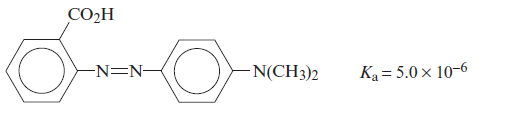# Problem: Methyl red has the following structure:It undergoes a color change from red to yellow as a solution gets more basic. Calculate an approximate pH range for which methyl red is useful. What is the color change and the pH at the color change when a weak acid is titrated with a strong base using methyl red as an indicator? What is the color change and the pH at the color change when a weak base is titrated with a strong acid using methyl red as an indicator? For which of these two types of titrations is methyl red a possible indicator?

###### FREE Expert Solution

General Reaction for an indicator:

HIn(aq) + H2O(l) ⇌ H3O+(aq) + In-(aq)

The pH range is as follow:

$\overline{){\mathbf{pH}}{\mathbf{=}}{{\mathbf{pK}}}_{{\mathbf{a}}}{\mathbf{±}}{\mathbf{1}}}$

Recall that pKa = -log Ka

${\mathbf{pH}}{\mathbf{=}}{\mathbf{-}}{\mathbf{log}}\left(5.0×{10}^{-6}\right){\mathbf{±}}{\mathbf{1}}\phantom{\rule{0ex}{0ex}}\phantom{\rule{0ex}{0ex}}{\mathbf{pH}}{\mathbf{=}}{\mathbf{5}}{\mathbf{.}}{\mathbf{3}}{\mathbf{±}}{\mathbf{1}}$

96% (70 ratings)###### Problem Details

Methyl red has the following structure:It undergoes a color change from red to yellow as a solution gets more basic. Calculate an approximate pH range for which methyl red is useful. What is the color change and the pH at the color change when a weak acid is titrated with a strong base using methyl red as an indicator? What is the color change and the pH at the color change when a weak base is titrated with a strong acid using methyl red as an indicator? For which of these two types of titrations is methyl red a possible indicator?

Frequently Asked Questions

What scientific concept do you need to know in order to solve this problem?

Our tutors have indicated that to solve this problem you will need to apply the Acid Base Indicators concept. If you need more Acid Base Indicators practice, you can also practice Acid Base Indicators practice problems.

What professor is this problem relevant for?

Based on our data, we think this problem is relevant for Professor Beren's class at UCLA.

What textbook is this problem found in?

Our data indicates that this problem or a close variation was asked in Chemistry: An Atoms First Approach - Zumdahl Atoms 1st 2nd Edition. You can also practice Chemistry: An Atoms First Approach - Zumdahl Atoms 1st 2nd Edition practice problems.